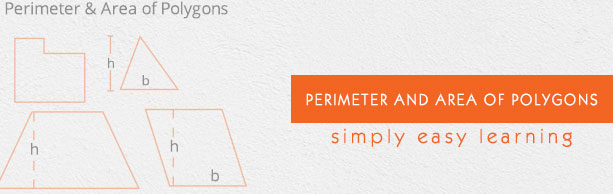# Perimeter and Area of Polygons

This tutorial provides comprehensive coverage of Perimeter and Area of Polygons based on Common Core (CCSS) and State Standards and its prerequisites. Students can navigate learning paths based on their level of readiness. Institutional users may customize the scope and sequence to meet curricular needs. This simple tutorial uses appropriate examples to help you understand Perimeter and Area of Polygons in a general and quick way.

# Audience

This tutorial has been prepared for beginners to help them understand the basics of Perimeter and Area of Polygons. After completing this tutorial, you will find yourself at a moderate level of expertise in Perimeter and Area of Polygons, from where you can advance further.

# Prerequisites

Before proceeding with this tutorial, you need a basic knowledge of elementary math concepts such as number sense, basic arithmetic operations, whole numbers, fractions, decimals, basic geometric concepts, 2d shapes, perimeters, areas, coordinate plane, and so on.